• 在已有的单链表中删除所有值为x元素，代码如下： class Node(object): # 初始化结点 def __init__(self,num): self.num=num self.next=None class SingleLinkedList(object): # 初始化头结点 def __init__...
• 请输入第1位元素：4 请输入第2位元素：1 请输入第3位元素：2 请输入第4位元素：3 请输入第5位元素：4 该线性表： 4 1 2 3 4 将值为4的元素删除后， 该线性表： 1 2 3 */ 创建单链表用到了...

算法思路定义两个指针r与p，其中让p指针始终在r指针的前面,即p = r->next 。让两个指针在链表中从头结点开始移动，如果p指针所指向结点的数据域的值与给定的值x相等,就要删除 。因为指针r指向该结点的前驱结点，所以我们可以轻松的进行删除操作

{
while (r->next != NULL)
//当指针r遍历整个链表后，退出while循环
{
if (p->data == (*x))
//判断指针p所指向的节点的数据域的值是否与x相等
{
r->next = p->next;
free (p);
}
//如果相等，则删除该节点
else
{
r = r->next;
}
//如果不相等，则指针继续移动
}

printf ("\n将值为%d的元素删除后，", (*x));
//将该链表中所有结点的数据域的值依次打印出来
}

用一个结点个数为4的单链表举例（头结点不存放元素）

它的原始形态：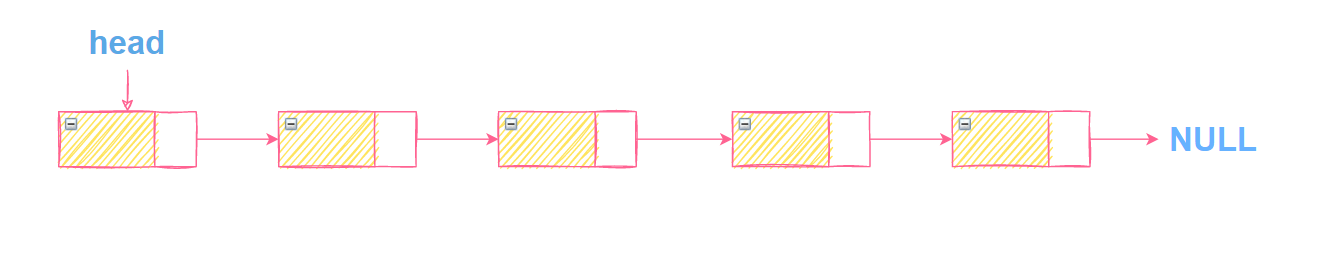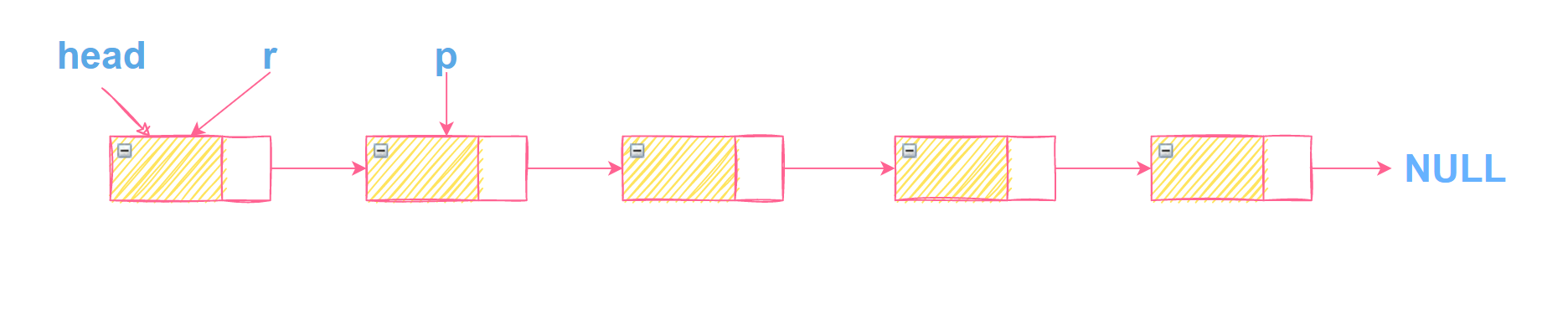当p->data == (*x)不成立时，指针r, p都要往后移动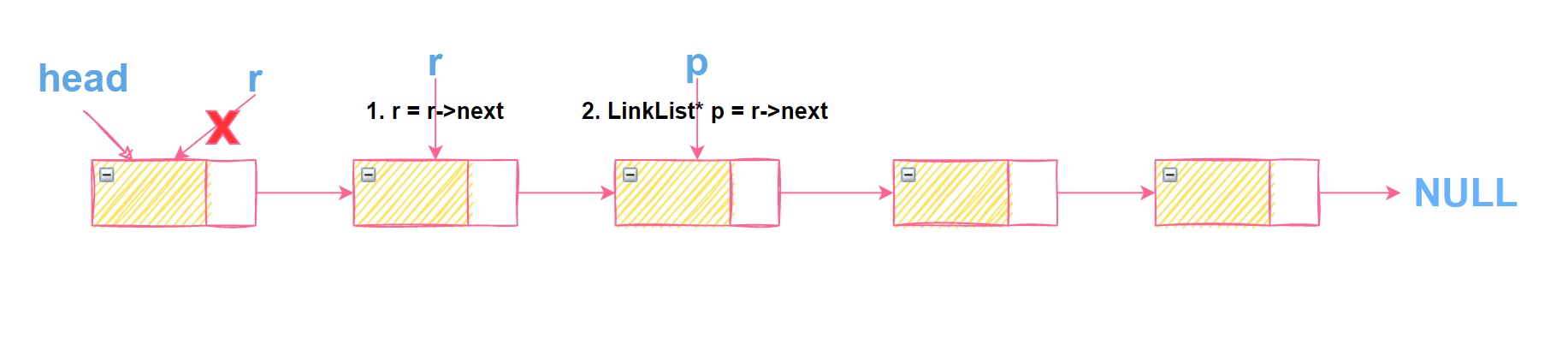当p->data == (*x)成立时，则要删除指针p所指向的结点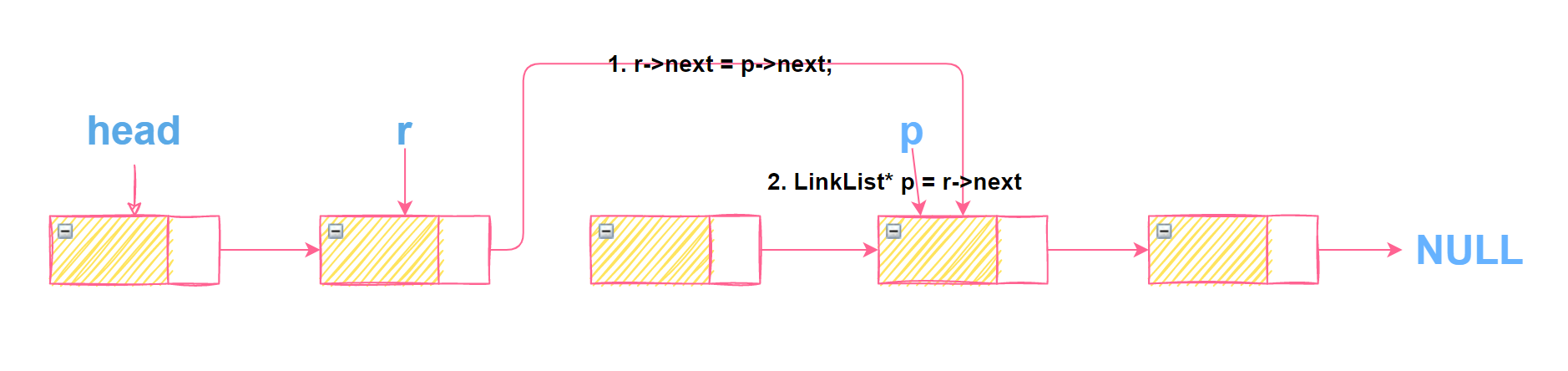=======================================
下面是完整的代码，用于验证我们的算法

#include <stdio.h>
#include <malloc.h>
//运用malloc函数需要添加该文件

typedef struct node
{
int data;
struct node* next;

//该函数的作用是将链表中各个元素的值依次打印出来
{
//给这个变量分配空间，r指向第一个节点的位置
printf ("\n该线性表为：");
while (r != NULL)
{
printf ("%5d", r->data);
r = r->next;
}
printf ("\n");
}

{
while (r->next != NULL)
//当指针r遍历整个链表后，退出while循环
{
if (p->data == (*x))
//判断指针p所指向的节点的数据域的值是否与x相等
{
r->next = p->next;
free (p);
//删除指针p所指向的结点
}
//如果相等，则删除该节点
else
{
r = r->next;
}
//如果不相等，则指针继续移动
}

printf ("\n将值为%d的元素删除后，", (*x));
//将该链表中所有结点的数据域的值依次打印出来
}

int main (void)
{
int i, r, len = 5;
//下面是尾插法创建单链表
for (i = 0; i < len; i++)
{
printf ("请输入第%d位元素的值：", i + 1);
scanf ("%d", &q->data);
q->next = NULL;
tail->next = q;
tail = q;
}

int x = 4;
return 0;
}
/*结果：
请输入第1位元素的值：4
请输入第2位元素的值：1
请输入第3位元素的值：2
请输入第4位元素的值：3
请输入第5位元素的值：4

该线性表为：    4    1    2    3    4

将值为4的元素删除后，
该线性表为：    1    2    3
*/

创建单链表用到了尾插法，如果对这个算法不够了解的小伙伴可以看看我写的另一篇文章，里面有详细的讲解

如果有更好的算法，欢迎小伙伴在评论区留言，一起交流学习！！！

展开全文算法 指针 链表 数据结构 单链表
• 删除所有值为x单链表中元素 首先，我们先考虑第一种情况，就是说值删除第一个值为x元素，这个比较简单， 只需要挨个比较x和链表节点的，找到相同的节点的前一个就可以删除这个节点了。 然后我们可以考虑...

删除所有值为x的单链表中的元素

首先，我们先考虑第一种情况，就是说值删除第一个值为x的元素，这个比较简单，

只需要挨个比较x和链表节点的值，找到值相同的节点的前一个就可以删除这个节点了。

然后我们可以考虑两种办法第一种就是递归的去删除，这个比较简单，只需要删除第一个值和我们要删除的值一样的节点，然后把下一个节点当做头指针传先去就好了。

这个就不给与代码了。我把头删的代码也贴上来。

{
{
delete pDelete;
pDelete = nullptr;
}
}

那么，我们来考虑一下非递归的方法吧。

PNODE DeleteByValue( PNODE* pHead, int val )
{
PNODE p1,p2;
PNODE pos;

{
}

{
while( p2 != NULL )
{
while( p2 != NULL && p2->val == val )
{
p1->next = p2->next;
delete p2;
p2 = NULL;
p2 = p1->next;
}
if(p2 == NULL )
{
break;
}
p1 = p1->next;
p2 = p2->next;
}
}

}

作为一个程序员应该是可以看懂这些代码的。首先，我们做了一个判断头结点的值是否和我们要删除的值相等，我们一直删除到第一个节点的值不和我们要删除的节点相等为止。首先，我们判断链表是否还有节点，如果有，我们维护两个指针，一个指向头结点，一个指向第二个节点，首先，这里有一个问题就是第一个指针指向的头结点一定不会被删除，因为前面已经删除了头结点的值一样的情况。所以我们在第二个指针不是空的前提下判断第二个节点的值是否和我们的值一样就可以了。如果，我们的第二个指针和要删除的值一样了那么删除第二个节点。首先，将第一的节点的下一个指向第二个节点的下一个。然后删除第二个节点。接着我们把第二个指针的值赋为第一的next，然后一直删除到第二个节点也不一样为止。这样就删除了一段值和我们要删除的相同的情况了。然后判断第二个指针是不是空了。空了 就退出。不空的后移。

展开全文• L, int x) //递归调用删除所有值为x元素 { LNode* p; if (L == NULL) return; if (L->data == x) { p = L; L = L->next; free(p); Del_x(L, x); } else Del_x(L->next, x); } 总体...

删除部分代码

void Del_x(LinkList& L, int x)      //递归调用删除所有值为x的元素
{
LNode* p;
if (L == NULL)
return;
if (L->data == x)
{
p = L;
L = L->next;
free(p);
Del_x(L, x);
}
else
Del_x(L->next, x);
}

总体代码测试

#include<stdlib.h>
#include<string.h>
#include<stdio.h>
typedef struct LNode
{
int data;
struct LNode* next;

{
LNode* s;
int x;
L->next = NULL;
printf("请输入链表元素的值（输入-1结束）\n:");
scanf_s("%d", &x);
while (x != -1)
{
s = (LNode*)malloc(sizeof(LNode));
s->next = NULL;
s->data = x;
s->next = L->next;
L->next = s;
scanf_s("%d", &x);
}
return L;
}
void Del_x(LinkList& L, int x)      //递归调用删除所有值为x的元素
{
LNode* p;
if (L == NULL)
return;
if (L->data == x)
{
p = L;
L = L->next;
free(p);
Del_x(L, x);
}
else
Del_x(L->next, x);
}
{
LNode* p;
p = L->next;                             //p指向第1号元素
if (p == NULL)
printf("NULL");
else
printf("打印链表中的元素:");

while (p != NULL)
{

printf("%d ", p->data);
p = p->next;

}
return L;
}

int main()
{
int x=0;
List_H(L);
printf("输入需要删除的元素:");
scanf_s("%d", &x);
Del_x(L, x);
PrintfList(L);
}

测试结果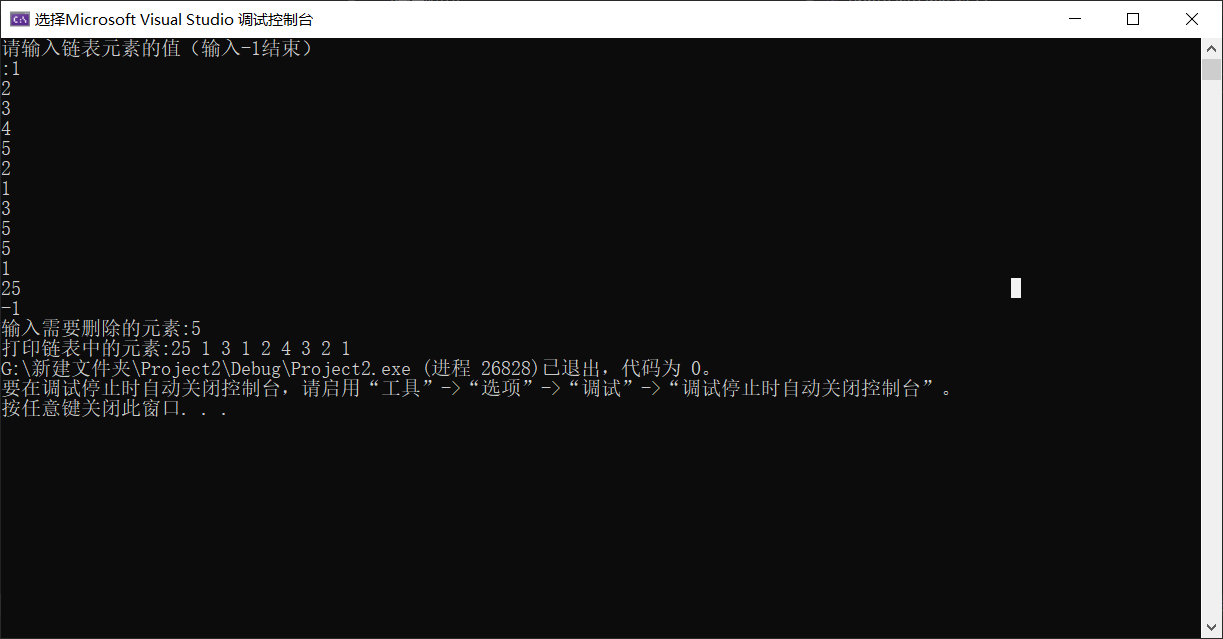展开全文链表 算法 数据结构 c语言 c++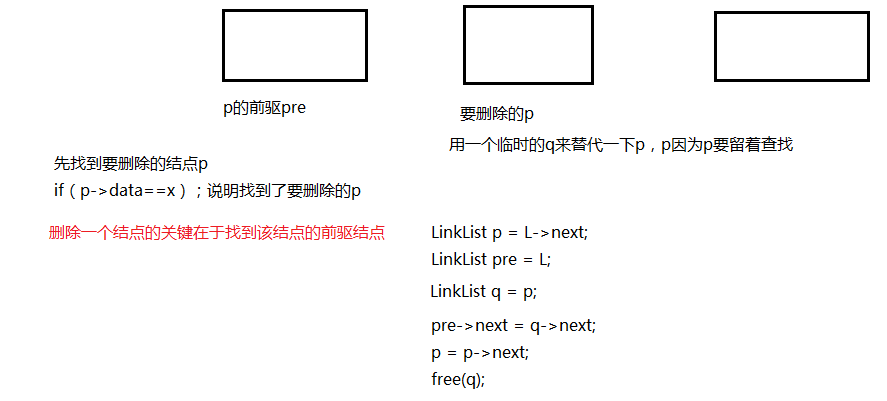#include<stdio.h>

#include<stdlib.h>

typedef struct LNode{
int data;
struct LNode *next;

p->next = NULL;
return p;
}

//带有头结点的单链表
int j = 1;//统计个数
if(i<0)
return NULL;
if(i==0)
return L;//返回头结点
while(p && j<i){
p = p->next;
j++;
}
return p;
}

//带有头结点的单链表
while(p && p->data!=e){
p = p->next;  //找不到就继续查找
}
return p;//找到就返回
}

int x;

scanf("%d",&x);
while(x!=9999){
s->data = x;
s->next = L->next;
L->next = s;
scanf("%d",&x);
}
return L;
}

int x;

scanf("%d",&x);
while(x!=9999){
s->data = x;
r->next = s;
r = s;
scanf("%d",&x);
}
r->next = NULL;
return L;

}

while(p){
printf("%d ",p->data);
p = p->next;
}
printf("\n");
}

s->data = num;
s->next = p->next;
p->next = s;

return L;

}

p->next = q->next;
free(q);

return L;
}

while(p!=NULL){
if(p->data == x){
q = p;
pre->next = q->next;
p = p->next;
free(q);

}
else{
pre = p;//关键pre要一直指向p的前驱
p = p->next;
}
}
return L;
}

int main(){

int x = 1;
int y = 0;
int num = 0;
scanf("%d%d",&x,&num);
while(x!=99 && num!=99){
Insert_Elem(L,x,num);
scanf("%d%d",&x,&num);
}
ListTraverse(L);

scanf("%d",&y);
Delete_SameX(L,y);
ListTraverse(L);
return 0;
}

展开全文• } void dele(LNode *L,int x) { LNode *p; if(L->next==NULL) return; else { dele(L->next,x); if(L->next->data==x) { p=L->next; L->next=p->next; free(p); } //dele(L->next,x); } ...
• L,int x){//用尾插法建立一个新的单链表，将为x元素链接到表尾 LNode *p=L->next,*r=L,*q;//假设单链表带头结点，p指针用来扫描整个链表，r指针初值头结点，之后始终指向表尾 while(p){ if(p->...
• 递归算法删除值为x的节点 本题苏州大学872考研真题（2010.3），现与大家讨论。 方法一 void Delete_x(LinkList &L,ElemType x){ if(L==NULL) return; else if(L->data==x){ p = L; L = L->next;...数据结构 单链表 递归算法
• 再带头节点的单链表L删除所有值为x的节点，并释放其空间，假设值为x的节点不唯一，试编写算法以实现上述操作。 二、分析 题目非常简单，只要熟悉单链表的基本操作就可以很快解决这个问题。 三、代码 #...链表 算法 数据结构
• 本人小白，所写东西完全练习数据结构，欢迎批评指正，若转载请注明出处，还望各位大佬指教，共同学习进步数据结构
• #include typedef struct node{ int data; struct node *next; }list,*nlist; nlist create() { nlist head=(nlist)malloc(sizeof(list)), end; // new =(nlist)malloc(sizeof(list)) ...int x=0; if
• 个人代码如下所示，欢迎大佬指正。 #include<stdio.h> #include<stdlib.h>...//删除值为x的结点 void Delete(Link& link, int x) { Node* p = link,*q; while (p->next!=NULL) {数据结构 单链表 链表
• 1.扫描单链表依次判断 void Del_x(LinkList &L,ElemType x) { LNode *p=L->next,*q=L,*s; while(p!=NULL) { if(p->data==x) { s=p; p=p->next; q->next=p; free(s); } ...单链表
•数据结构
• 单链表删除：通过图来看思路 p指针用来找链表数字为x的位置，pre指针始终指向p指针所指向位置的前一个位置最好自己在纸上模拟一下代码：#includeusing namespace std;typedef struct Node{int value;struct Node...
• 单链表删除元素时，可以按序号删除，也可以按数据删除。...L,int x){ //删除无头结点链表值为x的节点 LinkList p; //递归删除 if(L==NULL) return ; if(L->data==x){ p=L;...无头结点单链表
• 题目：设计一个递归算法，删除不带头结点的单链表中所有值为x的结点。 代码实现 //设计一个递归算法，删除不带头结点的单链表L中所有值为x的结点 #include"head.h"; typedef int Elemtype; //定义单链表 typedef ...链表 数据结构 单链表
• 原问题如下: 设计一个递归算法，删除不带头结点的单链表L中所有值为X的结点。答案使用递归法，算法如下：void Del_X(LinkList &amp;L, ElemType x){ LNode *P; if(L==NULL){ return; } if(L-&gt;data...
• 要求：设计一个算法，删除递增有序链表中值大于mink且小于maxk的所有元素（mink和maxk是给定的两个参数，其可以和表元素相同，也可以不同 ）。 [题目分析] 分别查找第一个>mink的结点和第一个 ≥maxk的...
• 项目任务在单链表增加一个功能erase(x y) 删除中所有值在 [x y] 之间的结点。假设链表结点数值类型是整形，要求编程返回删除相应结点后的链表长度和链表元素。输入描述第一行输入链表List的结点元素值元素...
• C语言 在带头结点的单链表中删除所有值为X的节点,并释放其空间 算法思想 因为L带头节点所以可以利用节点的前驱节点来删除节点,用*s遍历整个链表,当找到s的下一个节点为X值时,删除s的下一个节点 结构体 typedef ...算法 链表 数据结构
• public class node {//前一个节点private node prev;//后一个节点private node next;//private t value;public node() {super();}public node(node prev, node next, t value) {super();this.prev = prev;th...
• #include #include #include typedef int ElemType;... printf("删除小于xx="); scanf("%d",&x); DeleteListLessThanX(L,x); printf("数据展示"); PrintList(L); return 0; } 输出 输入 6 1 2 3 4 5 6 3链表 c语言 数据结构
• 方法一：public void deleteAllx1(int x){ Node p = head.next; Node q = head; while(p != null){ while(p != null && p.e != x){ q = p; p = p.n单链表 java
• 题目：在带头结点的单链表L删除所有值为x的结点，并释放其空间，假设值为x的结点不唯一，试编写算法实现上述操作。 算法思想：循环链表，标记待删除的结点x，并用p结点来覆盖标记x的结点，若循环到的结点不是x，...链表 数据结构
• 根据题目删除单链表L中所有值为X的结点，也就把if(data == x)的值删掉。本题的重点是考察递归算法，同时要求单链表不带头结点。 那么，下面具体看看使用递归算法是如何实现的。 一、递归算法代码 void Del_X...数据结构 C++
• 1、设计一个递归算法，删除不带头结点的单链表L中所有值为x的结点 答案如下 void Del_X_3(LinkList &L,ElemType x){ LNode *p; if(L==NULL) return ; if(L->data==x){ p=L; L=L->next; free(p); ...算法 链表 数据结构
• 1.设计一个递归算法,删除不带头结点的单链表中所有值为x的结点。 #include<iostream> #include<algorithm> #include<cstdio> #include<cstring> #include<cmath> #include<map...链表
• 方法一：用p从头至尾扫描单链表，pre指向*p结点的前驱。 代码如下： void Del_1(Linklist &L,ElemType x) { LNode *p=L->next,*pre=L,*q; while(p!=NULL) { if(p->data==x) { q=p; p=p->...数据结构 带头节点单链表 删除所有值为x的结点...